# C++ Programming

## C++ Programming

### Functions

Introduction

A Function is a sub-program that acts on data and often returns a value. Large programs are generally avoiding because it is difficult to manage a single list of instructions. Thus, a large program is broken down into smaller units known as functions. A functions is a named unit of a group of program statements. This unit can be invoked from other parts of the program.

### Why use Functions?

The most important reason to use functions is to make program handling easier as only a small part of the program is dealt with at a time, thereby avoiding ambiguity. Another reason to use functions is to reduce program size. Functions make a program more readable and understandable to a programmer thereby making program management much easier.

## C++ Function Types

There are two types of functions: library functions and user-defined functions. Library functions are predefined functions having their special meaning, whereas user-defined functions are the functions defined by the programmer/user. Library functions also called as built-in functions. You will learn in detail about function types in C++ Function Types tutorial.

## C++ Function Definition

In C++, a function must be defined before it is used anywhere in the program. You will learn more about function definition in C++ Function Prototype & Definition tutorial. But for now, let’s take some idea about it. Here is the general form to define a function in C++

```return_type function_name(comma_separated_parameter_list)
{
function body
}```

where return_type is any valid C++ data type specifies the type of value that the return statement of the function returns. In case, if no type is specified, the compiler assumed that the function returns an integer value. A function that doesn’t return any value is specified as void as their return_type. Now the function_name is any valid C++ identifier, which is the name of the function, and then comma_separated_parameter_list is the parameter list of the function separated by comma. Here is an example of a function definition in C++

```int sum(int a, int b)
{
}```

Or

```int sum(int a, int b)
{
return a+b;
}```

If a function doesn’t return any value, then specify return_type as void as shown in this example:

```void greeting(void)
{
cout<<"Hello C++";
}```

## C++ Function Prototype

A function prototype is a declaration of the function that tells the program about the type of the value returned by the function and the number and type of arguments. You will learn more about function prototype in C++ Function Prototype & Definition tutorial. But for now, let’s take some idea on it. Here is the general form:

`return_type function_name(parameter_list);`

Note semicolon here, as you saw in the above function definition, there is no semicolon. This can become a cause to differentiate function definition and function prototype in C++. Here is a function prototype example in C++

`int sum(int a, int b);`

You can also declare above as

`int sum(int, int);`

Since, function prototype only tells the compiler that the function sum has return type as int, and is declared having two parameters of integer types.

## Accessing a Function in C++

A function is called or invoked or executed, by providing the function name, followed by the parameters being sent enclosed in the parentheses. You will learn more about accessing a function in C++ Function Call tutorial. But for now, let’s take some idea about it. To invoke a function where prototype looks like as given above which was:

`int sum(int, int);`

then the function call statement may look like this:

`sum(a, b);`

where, a and b have to be int variables.

## C++ Function Examples

```/* C++ Functions - Example Program of C++ Functions
* This program demonstrates call by value method */

```#include<iostream.h>
#include<conio.h>
void swap(int, int);
void main()
{
clrscr();
int num1, num2;
cout<<"Enter any two number: ";
cin>>num1>>num2;
cout<<"\nBefore:\n\t";
cout<<"num1 = "<<num1<<"\tnum2 = "<<num2<<"\n\n";
swap(num1, num2);
cout<<"After:\n\t";
cout<<"num1 = "<<num1<<"\tnum2 = "<<num2<<"\n\n";
getch();
}
void swap(int a, int b)
{
int temp;
temp = a;
a = b;
b = temp;
cout<<"In:\n\t";
cout<<"num1 = "<<a<<"\tnum2 = "<<b<<"\n\n";
}``````

The above C++ program demonstrates the call by value method in C++. Here is the sample run of the above C++ program.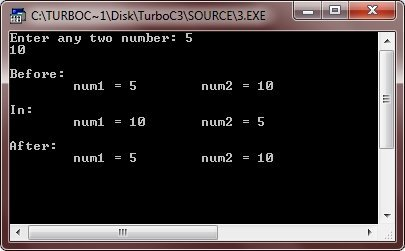Now this program demonstrates the call by reference method in C++

```/* C++ Functions - Example Program of C++ Functions
* This program demonstrates call by reference method */
```
#include<iostream.h>
#include<conio.h>
void swap(int &, int &);
void main()
{
clrscr();
int num1, num2;
cout<<"Enter any two number: ";
cin>>num1>>num2;
cout<<"\nBefore:\n\t";
cout<<"num1 = "<<num1<<"\tnum2 = "<<num2<<"\n\n";
swap(num1, num2);
cout<<"After:\n\t";
cout<<"num1 = "<<num1<<"\tnum2 = "<<num2<<"\n\n";
getch();
}
void swap(int &a, int &b)
{
int temp;
temp = a;
a = b;
b = temp;
cout<<"In:\n\t";
cout<<"num1 = "<<a<<"\tnum2 = "<<b<<"\n\n";
}``````

Since, call by reference method, operates on the address of the variables (argument passed). So value changed inside the function also affect the original value. Here is the sample run of the above C++ program.You can also write the above C++ program using pointers as shown here in this program.

```/* C++ Functions - Example Program of C++ Functions
* This program demonstrates call by reference method */
```
#include<iostream.h>
#include<conio.h>
void swap(int *, int *);
void main()
{
clrscr();
int num1, num2;
cout<<"Enter any two number: ";
cin>>num1>>num2;
cout<<"\nBefore:\n\t";
cout<<"num1 = "<<num1<<"\tnum2 = "<<num2<<"\n\n";
swap(&num1, &num2);
cout<<"After:\n\t";
cout<<"num1 = "<<num1<<"\tnum2 = "<<num2<<"\n\n";
getch();
}
void swap(int *a, int *b)
{
int temp;
temp = *a;
*a = *b;
*b = temp;
cout<<"In:\n\t";
cout<<"num1 = "<<*a<<"\tnum2 = "<<*b<<"\n\n";
}``````

Since, pointers are also operates on the memory address, so you can say the above program as call by reference program as shown in the second above program. Therefore the output produced by this C++ program is same as in the call by reference program shown above. Here is the output produced by this C++ program.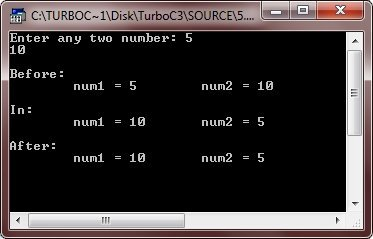Here is another type of C++ program also demonstrating functions.

```/* C++ Functions - Example Program of C++ Functions */

```#include<iostream.h>
#include<conio.h>
#include<stdlib.h>
int find_pos(char str[], char ch);
void main()
{
clrscr();
char strng, chr;
int y = 0;
cout<<"Enter the main string: ";
cin.getline(strng, 80);
cout<<"Enter a character to be searched for: ";
cin.get(chr);
y = find_pos(strng, chr);
if(y == -1)
{
cout<<"Press any key to exit..\n";
getch();
exit(1);
}
getch();
}
int find_pos(char str[], char ch)
{
int flag = -1, i, count=0;
for(i=0; str[i] != '\0'; i++)
{
if(str[i] == ch)
{
if(count>0)
{
cout<<", "<<i+1;
}
else
{
count++;
cout<<"\nThe character is in the string at position\n"<<i+1;
}
flag = 0;
}
}
return flag;
}``````

Below are the two sample runs of the above C++ program: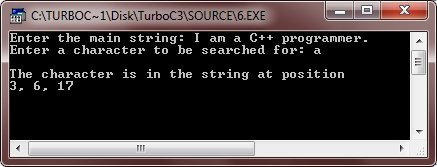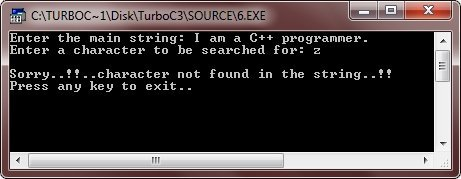Let’s take another program of C++ function.

```/* C++ Functions - Example Program of C++ Functions */

```#include<iostream.h>
#include<conio.h>
#include<stdlib.h>
void calculate(int, int, char);
void main()
{
clrscr();
int num1, num2;
char ch;
cout<<"Enter two numbers: ";
cin>>num1>>num2;
cout<<"Enter arithmetic operator (+, -, *, /, %): ";
cin>>ch;
calculate(num1, num2, ch);
getch();
}
void calculate(int a, int b, char c)
{
cout<<"\n";
switch(c)
{
case '+':
cout<<"Sum of "<<a<<" and "<<b<<" is "<<a+b;
break;
case '-':
cout<<"Difference of "<<a<<" and "<<b<<" is "<<a-b;
break;
case '*':
cout<<"Product of "<<a<<" and "<<b<<" is "<<a*b;
break;
case '/':
if(a<b)
{
cout<<"First number should be greater than second";
cout<<"\nPress any key to exit..\n";
getch();
exit(1);
}
cout<<"Quotient : "<<a<<" / "<<b<<" is "<<a/b;
break;
case '%':
if(a<b)
{
cout<<"First number should be greater than second\n";
cout<<"Press any key to exit...\n";
getch();
exit(2);
}
cout<<"Remainder : "<<a<<" % "<<b<<" is "<<a%b;
break;
default:
cout<<"Sorry..!!..Wrong Operator..!!\n";
cout<<"Press any key to exit..\n";
getch();
exit(3);
}
}``````

Below are some sample runs of this C++ program: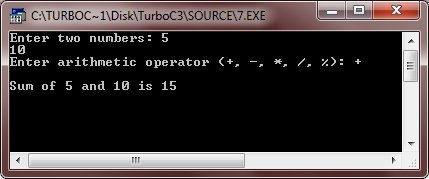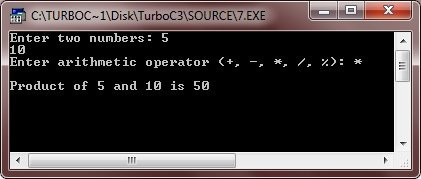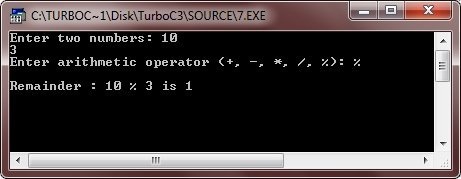Now it’s time to do it yourself and feel free to mess around with the program to understand in-depth what each statement does. Don’t forget to save your program.

That’s it for today, see you next week!!!